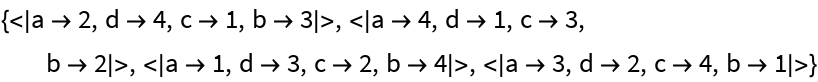#Function Repository Resource:

# FindOrderedHypergraphIsomorphism

Find isomorphisms between two ordered (directed) hypergraphs

Contributed by: Jonathan Gorard
 ResourceFunction["FindOrderedHypergraphIsomorphism"][h1,h2] finds all possible isomorphisms that map the ordered hypergraph h1 to h2 by renaming vertices.

## Details and Options

Hypergraphs are specified as lists of edges.
ResourceFunction["FindOrderedHypergraphIsomorphism"] gives an association of the form Association[v1{w1,},v2{w2,}], where vi are vertices in h1 and wj are corresponding vertices in h2.
ResourceFunction["FindOrderedHypergraphIsomorphism"] gives an empty association if no isomorphism can be found.
Two ordered hypergraphs are isomorphic if there exists a renaming of vertices that makes them identical.
The algorithm used in ResourceFunction["FindOrderedHypergraphIsomorphism"] is a direct generalization of Gorard’s uniqueness tree algorithm for standard graph isomorphism: https://arxiv.org/abs/1606.06399.

## Examples

### Basic Examples

Find all isomorphisms between two ordered hypergraphs:

 In:=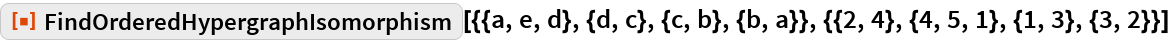Out=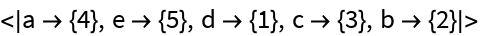Determine that two ordered hypergraphs are not isomorphic:

 In:=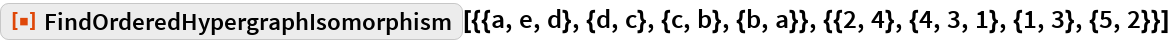Out=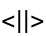Lists of hyperedges that are isomorphic as orderless hypergraphs may not be isomorphic as ordered hypergraphs:

 In:=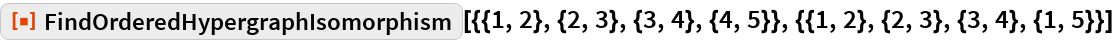Out=### Scope

FindOrderedHypergraphIsomorphism also works for standard directed graphs:

 In:=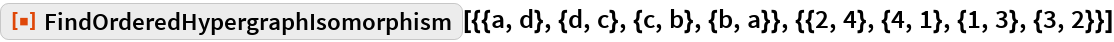Out=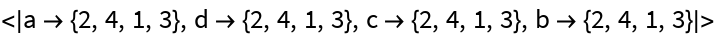In this case, it is functionally equivalent to :

 In:=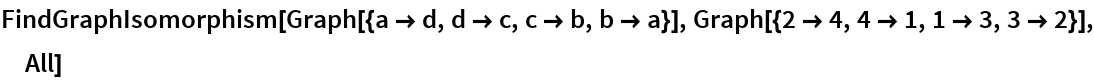Out=